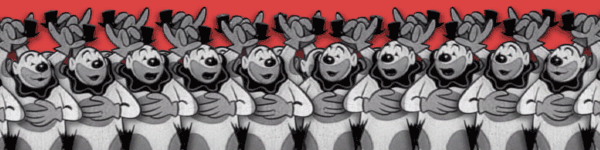# The five clownsFive clowns are planning for a trip to Los Angeles.They have to pack their things,including their collection of circles. Each clown uses a rectangular box and packs his/her circles linearly so that each circle touches the bottom of the box.

The clowns wants to spend as little as they can on the boxes so they buy ones with the smallest possible size. Given below is the radii of the circles each clown needs to pack.

$80,50,31,19,56,38,87,21$ $85,69,22,28,2,84,82,83$ $31,77,40,75,48,99,45$ $5,55,22,33,07$ $12,23,97,42,9,15,50,21$

Find the width of the smallest rectangular box each clown needs to buy and input the sum of the widths of the five rectangles as the answer.(rounded DOWN to the nearest integer).

Details/Assumptions

• Circles are arranged linearly and no circle is stacked on top of another.

• Size refers to the width or horizontal length of the smallest possible rectangle.

• Below is an example of an acceptable way of packing four circles,though it might not be the optimal way ;) .Explicit examples

• For three circles of radii $2,2,2$ the smallest possible size is $12$

• For $1,2,3$ it is $11.293$

• For $5,6$ it is $21.954$

×